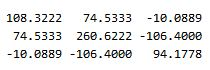2016-10-28 14:37:44 shenziheng1 阅读数 35399

# 2.拼出身—统计学的定义# 3.协方差矩阵的由来

1.协方差矩阵一定是个对称的方阵

2.协方差矩阵对角线上的因子其实就是变量的方差：cov(X,X)=var(X)# 3.MATLAB实战练习

MySample = fix(rand(10,3)*50)dim1 = MySample(:,1);
dim2 = MySample(:,2);
dim3 = MySample(:,3);


sum( (dim1-mean(dim1)) .* (dim2-mean(dim2)) ) / ( size(MySample,1)-1 ) % 得到  74.5333
sum( (dim1-mean(dim1)) .* (dim3-mean(dim3)) ) / ( size(MySample,1)-1 ) % 得到  -10.0889
sum( (dim2-mean(dim2)) .* (dim3-mean(dim3)) ) / ( size(MySample,1)-1 ) % 得到  -106.4000


std(dim1)^2 % 得到   108.3222
std(dim2)^2 % 得到   260.6222
std(dim3)^2 % 得到   94.1778

 C11=108.3222 C12=74.5333 C13=-10.0889 C21=74.5333 C22=260.6222 C23=-106.4000 C31=-10.0889 C32=-106.4000 C33=94.1778# 4.心得感悟

2016-06-19 16:30:21 cwcww1314 阅读数 2986

http://pinkyjie.com/2011/02/24/covariance-pca/

### PCA的缘起

PCA大概是198x年提出来的吧，简单的说，它是一种通用的降维工具。在我们处理高维数据的时候，为了能降低后续计算的复杂度，在“预处理”阶段通常要先对原始数据进行降维，而PCA就是干这个事的。本质上讲，PCA就是将高维的数据通过线性变换投影到低维空间上去，但这个投影可不是随便投投，要遵循一个指导思想，那就是：找出最能够代表原始数据的投影方法。这里怎么理解这个思想呢？“最能代表原始数据”希望降维后的数据不能失真，也就是说，被PCA降掉的那些维度只能是那些噪声或是冗余的数据。这里的噪声和冗余我认为可以这样认识：

• 噪声：我们常说“噪音污染”，意思就是“噪声”干扰我们想听到的真正声音。同样，假设样本中某个主要的维度A，它能代表原始数据，是“我们真正想听到的东西”，它本身含有的“能量”(即该维度的方差，为啥？别急，后文该解释的时候就有啦~)本来应该是很大的，但由于它与其他维度有那么一些千丝万缕的相关性，受到这些个相关维度的干扰，它的能量被削弱了，我们就希望通过PCA处理后，使维度A与其他维度的相关性尽可能减弱，进而恢复维度A应有的能量，让我们“听的更清楚”！
• 冗余：冗余也就是多余的意思，就是有它没它都一样，放着就是占地方。同样，假如样本中有些个维度，在所有的样本上变化不明显(极端情况：在所有的样本中该维度都等于同一个数)，也就是说该维度上的方差接近于零，那么显然它对区分不同的样本丝毫起不到任何作用，这个维度即是冗余的，有它没它一个样，所以PCA应该去掉这些维度。

### 协方差矩阵——PCA实现的关键

X={X1,,XN}Xi=(xi1,,xid)Rd,i=1,,NX={X1,…,XN}Xi=(xi1,…,xid)∈Rd,i=1,…,N

C=STSN1CRd×dC=STSN−1C∈Rd×d

ST1S1N1=Λ1S1TS1N−1=Λ1

PTCP=ΛPT1CP1=Λ1PTCP=Λ⇒P1TCP1=Λ1

ST1S1N1=Λ1=PT1CP1=PT1(STSN1)P1=(SP1)T(SP1)N1S1TS1N−1=Λ1=P1TCP1=P1T(STSN−1)P1=(SP1)T(SP1)N−1

S1=SP1S1RN×p⇒S1=SP1S1∈RN×p

1. 形成样本矩阵，样本中心化
1. 计算样本矩阵的协方差矩阵
1. 对协方差矩阵进行特征值分解，选取最大的p个特征值对应的特征向量组成投影矩阵
1. 对原始样本矩阵进行投影，得到降维后的新样本矩阵

### Matlab中PCA实战

 1 S = fix(rand(10,3)*50);1234 S = S - repmat(mean(S),10,1);C = (S'*S)./(size(S,1)-1);orC = cov(S);1 [P,Lambda] = eig(C);1 S1 = S*P;1 [COEFF,SCORE] = princomp(S) % COEFF表示投影矩阵，SCORE表示投影后新样本矩阵2014-08-31 12:30:00 weixin_34375054 阅读数 192

所以，按照定义，给定的4个二维样本的协方差矩阵为：

z=[1,2;3,6;4,2;5,2]

cov(z)

ans =

2.9167   -0.3333

-0.3333    4.0000

协方差(i,j)=（第i列所有元素-第i列均值）*（第j列所有元素-第j列均值）/（样本数-1

下面在给出一个4维3样本的实例，注意4维样本与符号X,Y就没有关系了，X,Y表示两维的，4维就直接套用计算公式，不用X,Y那么具有迷惑性的表达了。

（3）与matlab计算验证

Z=[1 2 3 4;3 4 1 2;2 3 1 4]

cov(Z)

ans =

1.0000    1.0000   -1.0000   -1.0000

1.0000    1.0000   -1.0000   -1.0000

-1.0000   -1.0000    1.3333    0.6667

-1.0000   -1.0000    0.6667    1.3333

可知该计算方法是正确的。我们还可以看出，协方差矩阵都是方阵，它的维度与样本维度有关（相等）。参考2中还给出了计算协方差矩阵的源代码，非常简洁易懂，在此感谢一下!

 http://en.wikipedia.org/wiki/Covariance_matrix

 http://www.cnblogs.com/cvlabs/archive/2010/05/08/1730319.html

http://blog.csdn.net/ybdesire/article/details/6270328

2018-10-22 21:29:22 u010420283 阅读数 474

### 0. 前言

MATLAB或者OpenCV里有很多封装好的函数，我们可以使用一行代码直接调用并得到处理结果。然而当问到具体是怎么实现的时候，却总是一脸懵逼，答不上来。前两天参加一个算法工程师的笔试题，其中就考到了这几点，感到非常汗颜！赶紧补习！

### 1. 双线性插值tra_im[10,4]=ori_im[x1,y1]*(17-16.67）*（7-6.67）+ori_im[x2,y1]*(16.67-16）*（6.67-6）+ori_im[x2,y1]*(17-16.67）*（6.67-6）+ori_im[x2,y2]*(16.67-16）*（6.67-6）

# -*- coding: utf-8 -*-
# Author: lmh
# Time: 2018.10.22
from skimage import transform
import matplotlib.pyplot as plt
import matplotlib.image as mping
import numpy as np
def chazhi(x,y,im)：
#x,y分别是缩放或者放大后对应源图像的浮点坐标位置，im是源图像，返回目标图像根据插值计算得到的像素值
x1,y1=int(x),int(y) #x1,x2,x3,x4分别是插值坐标对应在源图像上下左右最近的点的坐标
x2,y2=x1+1,y1+1
pixel11,pixel21,pixel12,pixel22=im[x1-1,y1-1],im[x2-1,y1-1],im[x2-1,y1-1],im[x2-1,y2-1]
#以下是根据双线性插值的公式求得的目标图像的该位置的像素值
new_pixel=(x2-x)*(y2-y)*pixel11+(x-x1)*(y2-y)*pixel21+(x2-x)*(y-y1)*pixel12+(x-x1)*(y-y1)*pixel22
return new_pixel

im11=im
scale=0.4 #缩小程度
row,col=int(im.shape*scale),int(im.shape*scale)
im_sml=np.zeros([row,col,3])
for k in range(3):
im1 = im[:, :, k]
for i in range(row):
for j in range(col):
value=chazhi(i/scale,j/scale,im1)#3通道图像逐像素计算缩小或者放大后的新像素值
im_sml[i][j][k]=value

im_narrow=im_sml

scale=1.7  #扩大程度
row, col = int(im.shape * scale), int(im.shape * scale)
im_sml = np.zeros([row, col, 3])
for k in range(3):
im1 = im[:, :, k]
for i in range(row):
for j in range(col):
value = chazhi(i / scale, j / scale, im1)
im_sml[i][j][k] = value

im_enlarge=im_sml
python_narrow=transform.resize(im, (175, 145)) #使用skimage自带函数resize图像，也可以直接写像上面一样写比例（0.4,1.7）
python_enlarge=transform.resize(im, (746, 617))#为了对比，两种方法特意放大和缩小一样大小

plt.figure()
plt.subplot(151)
plt.imshow(im11,plt.cm.gray)
plt.title('Original')
# plt.axis('off')

plt.subplot(152)
plt.imshow(im_narrow,plt.cm.gray)
plt.title('my_narrow')
# plt.axis('off')

plt.subplot(153)
plt.imshow(python_narrow,plt.cm.gray)
plt.title('skimage_narrow')
# plt.axis('off')

plt.subplot(154)
plt.imshow(im_enlarge,plt.cm.gray)
plt.title('my_enlarge')
# plt.axis('off')

plt.subplot(1,5,5)
plt.imshow(python_enlarge,plt.cm.gray)
plt.title('skimage_enlarge')
# plt.axis('off')
plt.savefig('image_comp.png')

skimage的源码：

效果图对比（原图[439,363,3]；缩小0.4倍后为[175,145,3]；放大1.7倍后为[746,617,3]）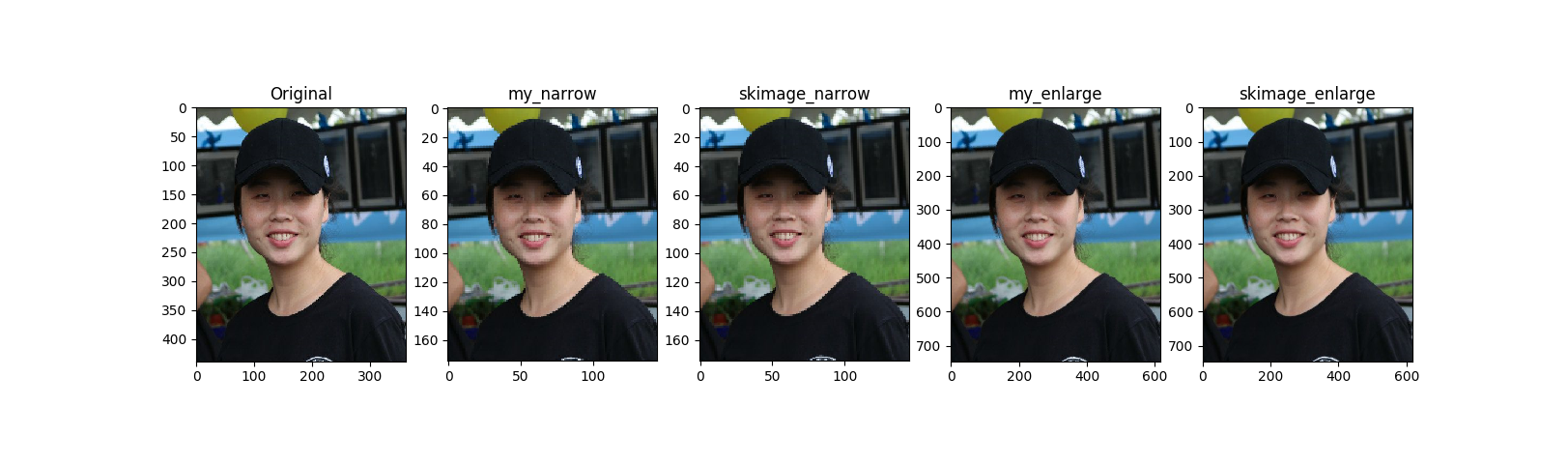int x=(i+0.5)*m/a-0.5

int y=(j+0.5)*n/b-0.5

int x=i*m/a

int y=j*n/b

# take into account that 0th pixel is at position (0.5, 0.5)
dst_corners[:, 0] = col_scale * (src_corners[:, 0] + 0.5) - 0.5
dst_corners[:, 1] = row_scale * (src_corners[:, 1] + 0.5) - 0.5

### 3. 求矩阵的特征值，特征向量，以及主成分

2018-06-28 14:21:01 qq_40213457 阅读数 60

# 2.拼出身—统计学的定义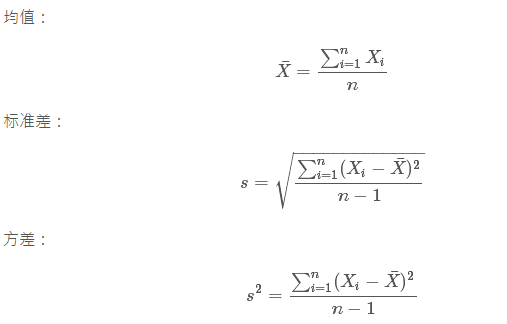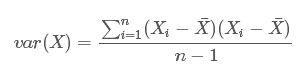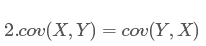# 3.协方差矩阵的由来

1.协方差矩阵一定是个对称的方阵

2.协方差矩阵对角线上的因子其实就是变量的方差：cov(X,X)=var(X)# 3.MATLAB实战练习

MySample = fix(rand(10,3)*50)1. dim1 = MySample(:,1);1列
2. dim2 = MySample(:,2);2列
3. dim3 = MySample(:,3);3列

1. sum( (dim1-mean(dim1)) .* (dim2-mean(dim2)) ) / ( size(MySample,1)-1 ) % 得到 74.5333
2. sum( (dim1-mean(dim1)) .* (dim3-mean(dim3)) ) / ( size(MySample,1)-1 ) % 得到 -10.0889
3. sum( (dim2-mean(dim2)) .* (dim3-mean(dim3)) ) / ( size(MySample,1)-1 ) % 得到 -106.4000

1. std(dim1)^2 % 得到 108.3222
2. std(dim2)^2 % 得到 260.6222
3. std(dim3)^2 % 得到 94.1778

 C11=108.3222 C12=74.5333 C13=-10.0889 C21=74.5333 C22=260.6222 C23=-106.4000 C31=-10.0889 C32=-106.4000 C33=94.1778